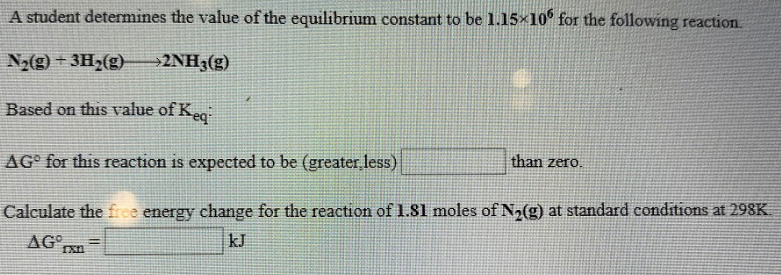# A student determines the value of the equilibrium constant to be 1.15x10^6 for the following reaction. N2(g) + 3H2(g) → 2NH3(g) Based on this value of Keq: ΔG° for this reaction is expected to be (greater, less) ____ than zero. Calculate the free energy change for the reaction of 1.81 moles of N2(g) at standard conditions at 298K. ΔG°rxn = ____ kJ.